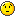# CINT auto completion in 5.28

in 5.28 the auto completion is not working with an object instance or pointer, but it works for a class qualifier. for example:

[code]root  TH1F h
root  h.Error in TTabCom::DetermineClass: variable “h” not defined?

problem determining class of “h”

variable “h.” not defined.
root  TH1F::
~TH1F
TH1F

[/code]

I don’t remember if 5.26 works but i think it should otherwise i’d posted then. I checked it’s not related with backtrace problem, and i have the editline option enabled when compiling root. I am running ubuntu 10.10.

somehow it works in my home pc (ubuntu10.10 too). but not in office pc…
5.26 doesn’t work in my office pc either…

one difference is home pc is running g++4.4.5, office is running 4.5.1

Hi,

I can not reproduce this problem. It is possible that it is an installation problem (check that ROOTSYS, PATH and LD_LIBRARY_PATH are consistent). Also you could try to see if the problem is related to uninitialized memory by trying with valgrind (valgrind root.exe -b -l )

Cheers,
Philippe.

ok here’s what i found out by reading the TTabCom.cxx DetermineClass member function. basically it determines the class of a variable (name) by output to a tmp file, for example, supposed i defined a TH1F h, when pressing the tab after “h.”, the code does the following:

``````gROOT->ProcessLine("h"); > /tmp/ttt
inf = ifstream("/tmp/ttt")``````

then by parsing the file content it gets the class name.

Now comes the really weird problem. Here I modified the source code to expose the probelm

``````   check = (!file1 || c <= 0 || c == '*' || c != '(');
/*if ((!file1 || c <= 0 || c == '*' || c != '(')) {*/ // this is the original line that caused the problem.
if (check) { // instead, using this line will fix the problem.
cout<<"DEBUG:: why are we here? "<<(!file1)<<", "<<(c <= 0)<<", "<<(c != '(')<<endl;
Error("TTabCom::DetermineClass", "variable \"%s\" not defined?",
varName);
goto cleanup;
}``````

if i use the commented out condition line, i have the problem. Even though a cout of check in front of it will show the check actually returned 0. Somehow the if-condition breaks? in g++4.5.1? Maybe there are some weird optimization problem?

Anyway I fixed my problem. Hope it works for anyone else having the same trouble.ok really weird. the above solution just worked once, now it failed again…maybe when the check or the if condition is evaluated, either the tmp file or c are not ready thus has some weird values??? but i don’t see there’s multiple thread/racing condition involved…

Anyway, the following code works for me more consistantly now:

``` check = (!file1) || c <= 0 || c == '*' || c != '('; if (check) { cout<<check<<"=yes: "<<(!file1)<<", "<<(c <= 0)<<", "<<(c != '(')<<" = "<<(!file1 || c <= 0 || c == '*' || c != '(')<<endl; check = (!file1) || c <= 0 || c == '*' || c != '('; cout<<"check again: "<<check<<", "<<(!file1)<<", "<<(c <= 0)<<", "<<(c != '(')<<" = "<<(!file1 || c <= 0 || c == '*' || c != '(')<<en\ dl; } else { cout<<check<<"=no"<<endl; } if (check) { cout<<"DEBUG:: why are we here? "<<(!file1)<<", "<<(c <= 0)<<", "<<(c != '(')<<endl; Error("TTabCom::DetermineClass", "variable \"%s\" not defined?", varName); goto cleanup; } ```

NOTE, if i remove the cout’s it will fail again. So for now i will leave them in. It’s not bothering me too much…

for example:

[code]root  TH1F f
root  f.1=yes: 0, 0, 0 = 0
check again: 0, 0, 0, 0 = 0

~TH1F
TH1F

[/code]

Hi,

[quote]NOTE, if i remove the cout’s it will fail again. So for now i will leave them in. It’s not bothering me too much…[/quote]This strongly points to a deficiency in the compiler (optimizer).

Cheers,
Philippe.